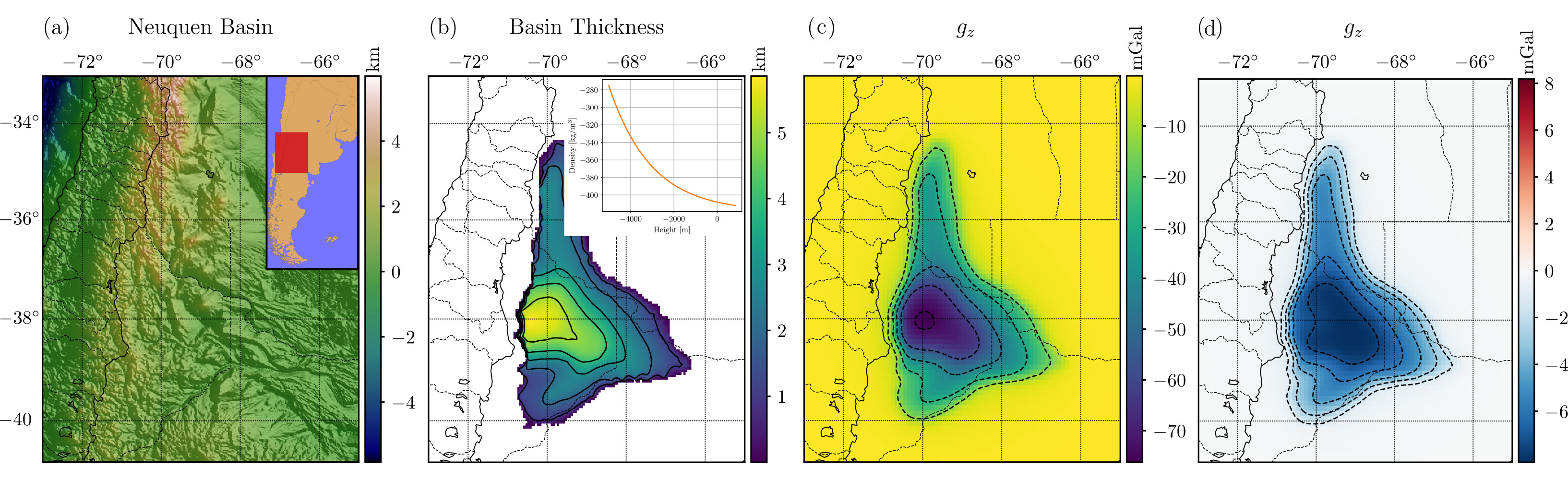# Gravitational field calculation in spherical coordinates using variable densities in depth

Santiago R. Soler, Agustina Pesce, Mario E. Giménez and Leonardo Uieda (2019)

## Abstract

We present a new methodology to compute the gravitational fields generated by tesseroids (spherical prisms) whose density varies with depth according to an arbitrary continuous function. It approximates the gravitational fields through the Gauss-Legendre Quadrature along with two discretization algorithms that automatically control its accuracy by adaptively dividing the tesseroid into smaller ones. The first one is a preexisting two dimensional adaptive discretization algorithm that reduces the errors due to the distance between the tesseroid and the computation point. The second is a new density-based discretization algorithm that decreases the errors introduced by the variation of the density function with depth. The amount of divisions made by each algorithm is indirectly controlled by two parameters: the distance-size ratio and the delta ratio. We have obtained analytical solutions for a spherical shell with radially variable density and compared them to the results of the numerical model for linear, exponential, and sinusoidal density functions. The heavily oscillating density functions are intended only to test the algorithm to its limits and not to emulate a real world case. These comparisons allowed us to obtain optimal values for the distance-size and delta ratios that yield an accuracy of 0.1% of the analytical solutions. The resulting optimal values of distance-size ratio for the gravitational potential and its gradient are 1 and 2.5, respectively. The density-based discretization algorithm produces no discretizations in the linear density case, but a delta ratio of 0.1 is needed for the exponential and most sinusoidal density functions. These values can be extrapolated to cover most common use cases, which are simpler than oscillating density profiles. However, the distance-size and delta ratios can be configured by the user to increase the accuracy of the results at the expense of computational speed. Lastly, we apply this new methodology to model the Neuquén Basin, a foreland basin in Argentina with a maximum depth of over 5000m, using an exponential density function.Application of the methodology to the Neuquén Basin, a foreland basin in the southern Andes. (a) Topography of the Neuquén Basin and its location in South America. (b) Thickness of the sedimentary basin. Inset shows the exponential density profile used in the modeling. (c) Resulting vertical gravitational acceleration at 10 km height modeled by tesseroids with exponential density variation in depth. (d) Difference between gravitational acceleration modeled using the exponential density profile and a homogeneous density.

## How to cite

Soler, S. R., Pesce, A., Gimenez, M. E., & Uieda, L., 2019. Gravitational field calculation in spherical coordinates using variable densities in depth, Geophysical Journal International. doi: 10.1093/gji/ggz277